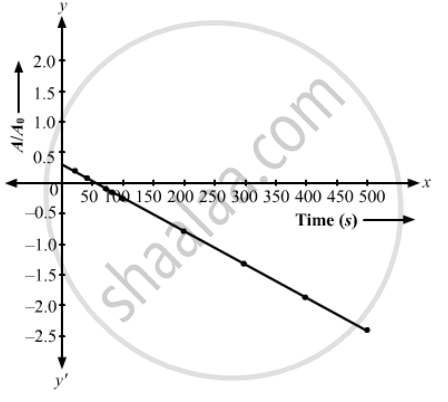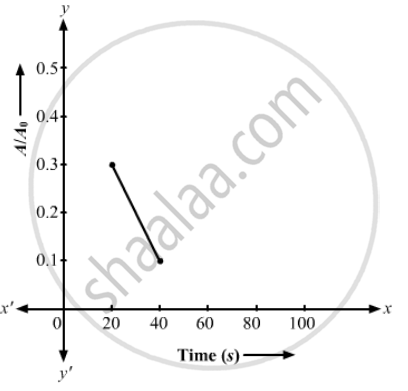Department of Pre-University Education, KarnatakaPUC Karnataka Science Class 12
Share

# A Sample Contains a Mixture of 108ag and 110ag Isotopes Each Having an Activity of 8.0 × 108 Disintegration per Second. 110ag is Known to Have Larger Half-life than 108ag. - Physics

#### Question

A sample contains a mixture of 108Ag and 110Ag isotopes each having an activity of 8.0 × 108 disintegration per second. 110Ag is known to have larger half-life than 108Ag. The activity A is measured as a function of time and the following data are obtained.

 Time (s) Activity (A)(108 disinte-grations s−1) Time (s) Activity (A108 disinte-grations s−1) 20406080100 11.7999.16807.44926.26845.4115 200300400500 3.08281.88991.16710.7212

(a) Plot ln (A/A0) versus time. (b) See that for large values of time, the plot is nearly linear. Deduce the half-life of 110Ag from this portion of the plot. (c) Use the half-life of 110Ag to calculate the activity corresponding to 108Ag in the first 50 s. (d) Plot In (A/A0) versus time for 108Ag for the first 50 s. (e) Find the half-life of 108Ag.

#### Solution

(a) Activity, A0 = 8 × 108 dis/sec

(i) "In" (A_1/A_0) = "In" (11.794/8) = 0.389

(ii) "In" (A_2/A_0) = "In" (9.1680/8) = 0.12362

(iii) "In" (A_3/A_0) = "In" (7.4492/8) = -0.072

(iv) "In" (A_4/A_0) = "In" (6.2684/6) = -0.244

(v) "In" (A_5/A) = "In" (5.4115/8) = -0.391

(vi) "In" (A_6/A_0) = "In" (3.0828/8) = -0.954

(vii) "In" (A_7/A_0) = "In" (91.8899/8) = -1.443

(viii) "In" (1.1671/8) = "In" (90.7212/8) = -1.93

(ix) "In" (0.7212/8) = "In" (90.7212/8) = -2.406

The required graph is given below.(b) Half-life of 110Ag = 24.4 s
(c) Half-life of 110Ag,  T_"1/2"= 24.4 s

Decay constant, lambda = 0.693/T_"1/2"

⇒ lambda = 0.63/24.4 = 0.0284

therefore t = 50 sec

Activity , "A" = "A"_0e^(-lambdat)

= 8 xx 10^8 xx e^(-0.0284 xx 50)

= 1.93 xx 10^8

(d)(e) The half-life period of ""^108"Ag" that you can easily watch in your graph is 144 s .

Is there an error in this question or solution?

#### APPEARS IN

Solution A Sample Contains a Mixture of 108ag and 110ag Isotopes Each Having an Activity of 8.0 × 108 Disintegration per Second. 110ag is Known to Have Larger Half-life than 108ag. Concept: Radioactivity - Introduction of Radioactivity.
S